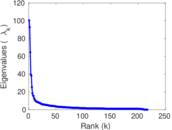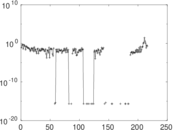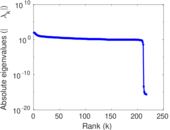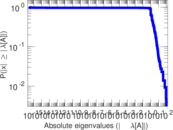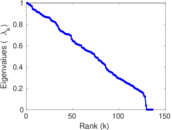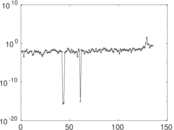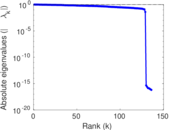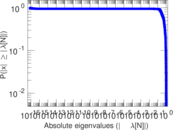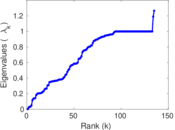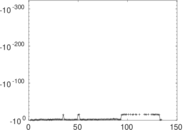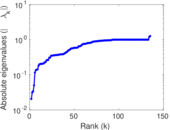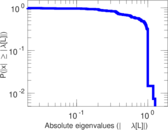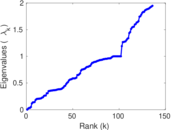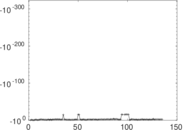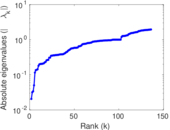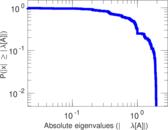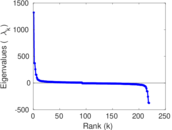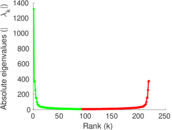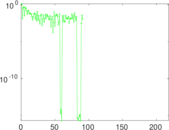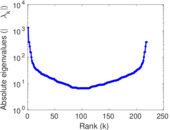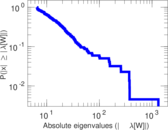# Wikiquote edits (ku)

This is the bipartite edit network of the Kurdish Wikiquote. It contains users and pages from the Kurdish Wikiquote, connected by edit events. Each edge represents an edit. The dataset includes the timestamp of each edit.

 Code `qku` Internal name `edit-kuwikiquote` Name Wikiquote edits (ku) Data source http://dumps.wikimedia.org/ AvailabilityDataset is available for download Consistency checkDataset passed all tests Category Authorship network Dataset timestamp 2017-10-20 Node meaning User, article Edge meaning Edit Network formatBipartite, undirected Edge typeUnweighted, multiple edges Temporal dataEdges are annotated with timestamps

## Statistics

 Size n = 1,333 Left size n1 = 219 Right size n2 = 1,114 Volume m = 4,929 Unique edge count m̿ = 2,731 Wedge count s = 175,482 Claw count z = 13,182,431 Cross count x = 961,834,975 Square count q = 136,027 4-Tour count T4 = 1,799,198 Maximum degree dmax = 915 Maximum left degree d1max = 915 Maximum right degree d2max = 136 Average degree d = 7.395 35 Average left degree d1 = 22.506 8 Average right degree d2 = 4.424 60 Fill p = 0.011 194 2 Average edge multiplicity m̃ = 1.804 83 Size of LCC N = 1,029 Diameter δ = 13 50-Percentile effective diameter δ0.5 = 3.618 38 90-Percentile effective diameter δ0.9 = 5.852 49 Median distance δM = 4 Mean distance δm = 4.282 84 Gini coefficient G = 0.777 434 Balanced inequality ratio P = 0.180 361 Left balanced inequality ratio P1 = 0.131 264 Right balanced inequality ratio P2 = 0.233 922 Relative edge distribution entropy Her = 0.818 843 Power law exponent γ = 2.639 13 Tail power law exponent γt = 1.961 00 Tail power law exponent with p γ3 = 1.961 00 p-value p = 0.000 00 Left tail power law exponent with p γ3,1 = 1.641 00 Left p-value p1 = 0.370 000 Right tail power law exponent with p γ3,2 = 8.981 00 Right p-value p2 = 0.933 000 Degree assortativity ρ = −0.026 374 3 Degree assortativity p-value pρ = 0.168 234 Spectral norm α = 100.435 Algebraic connectivity a = 0.020 533 0 Spectral separation |λ1[A] / λ2[A]| = 1.082 87 Controllability C = 891 Relative controllability Cr = 0.678 599

## Plots

### Fruchterman–Reingold graph drawing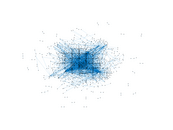### Degree distribution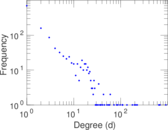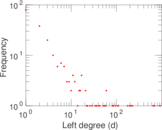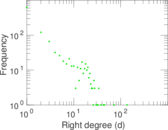### Cumulative degree distribution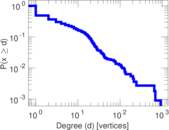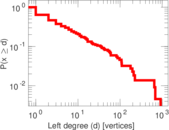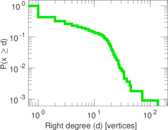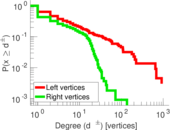### Lorenz curve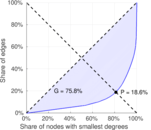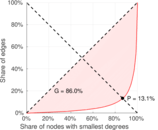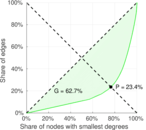### Spectral distribution of the adjacency matrix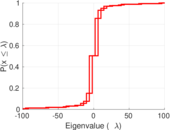### Spectral distribution of the normalized adjacency matrix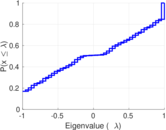### Spectral distribution of the Laplacian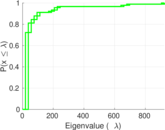### Spectral graph drawing based on the adjacency matrix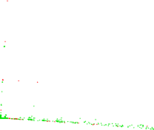### Spectral graph drawing based on the Laplacian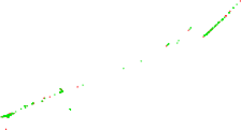### Spectral graph drawing based on the normalized adjacency matrix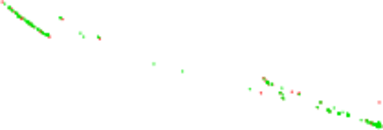### Degree assortativity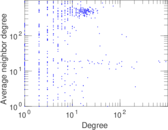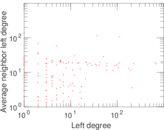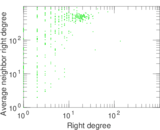### Zipf plot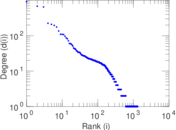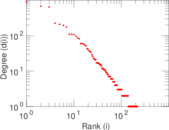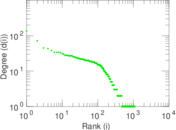### Hop distribution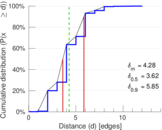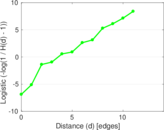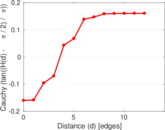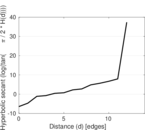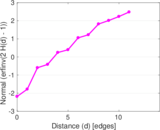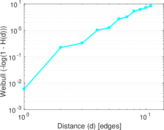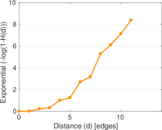### Double Laplacian graph drawing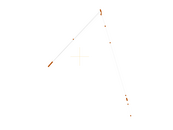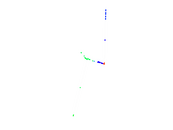### Delaunay graph drawing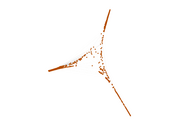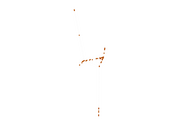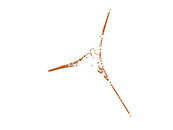### Edge weight/multiplicity distribution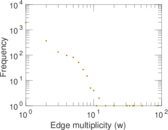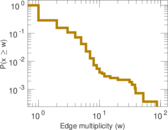### Temporal distribution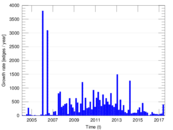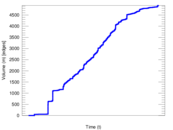### Temporal hop distribution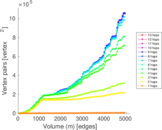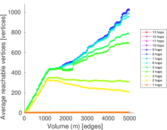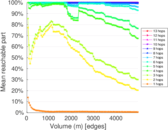### Diameter/density evolution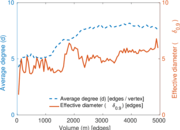### Matrix decompositions plots